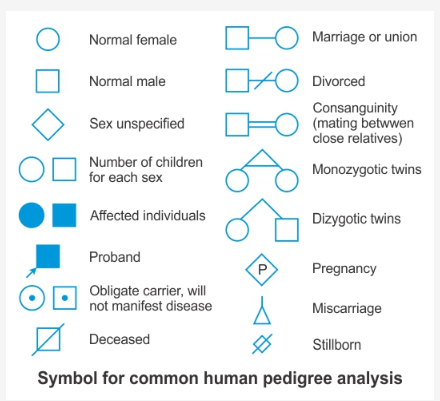# Please give me the format of Biology Practical Exam

Please give me the format of Biology Practical Exam.
Please explain how to write the perfect answers to the following questions:

1. Identify the Pedigree chart.
2. Determine the pH of given soil samples.
3. Find out the Mendelian ratio.

There will be two 5 mark question on writing a particular experiment (inclusive of aim, materials required etc). One question of 4 marks will be to perform a particular experiment and writing down the results. Spotting questions carry 6 marks (can be 2 or 3). Rest 10 questions will be divided into practical manual and viva voce.

1. A pedigree chart can be identified if the symbols can be remembered. For example, squares represent males. Circles are females. Horizontal lines between square and circles represent husband and wife. A darkened shape is an affected individual - male or female. A hollow shape is a normal individual.For example, if the trait is observed in generation-I and not in generation-II, but re-appears in subsequent generations, then the trait is “recessive”. So, for recessive characters, the pedigree is shown below2. The soil pH reflects whether a soil is acidic, neutral, basic or alkaline. The acidity, neutrality or alkalinity of a soil is measured in terms of hydrogen ion activity of the soil water system.
Determination of pH using pH Paper

• Take a pH paper strip and place it on a white tile.
• Pour a drop of the sample on the pH paper using a clean dropper.
• Observe the colour of the pH paper.
• Now compare the colour obtained on the pH paper with the different colour shades of the standard colour pH chart and note down the pH value.
• Similarly, find the pH of the remaining samples using a fresh strip of pH paper and a separate dropper for each sample
1. Mendelian ratios can be calculated by punette square. For example, to calculate phenotypic ratioFill in the Punnet square by crossing one column and row and writing the result in each square. So a cross of “A” and “a” should be written as “Aa.” Write the amount of homozygous dominant (AA) and heterozygous (Aa) squares as one phenotypic group. Count the amount of homozygous recessive (aa) squares as another group. Write the result as a ratio of the two groups. A count of 3 from one group and 1 from the other would give a ratio of 3:1.